University Physics Volume 2

# Problems

### 6.1Electric Flux

20.

A uniform electric field of magnitude $1.1×104N/C1.1×104N/C$ is perpendicular to a square sheet with sides 2.0 m long. What is the electric flux through the sheet?

21.

Calculate the flux through the sheet of the previous problem if the plane of the sheet is at an angle of $60°60°$ to the field. Find the flux for both directions of the unit normal to the sheet.

22.

Find the electric flux through a rectangular area $3cm×2cm3cm×2cm$ between two parallel plates where there is a constant electric field of 30 N/C for the following orientations of the area: (a) parallel to the plates, (b) perpendicular to the plates, and (c) the normal to the area making a $30°30°$ angle with the direction of the electric field. Note that this angle can also be given as $180°+30°.180°+30°.$

23.

The electric flux through a square-shaped area of side 5 cm near a large charged sheet is found to be $3×10−5N·m2/C3×10−5N·m2/C$ when the area is parallel to the plate. Find the charge density on the sheet.

24.

Two large rectangular aluminum plates of area $150cm2150cm2$ face each other with a separation of 3 mm between them. The plates are charged with equal amount of opposite charges, $±20μC±20μC$. The charges on the plates face each other. Find the flux through a circle of radius 3 cm between the plates when the normal to the circle makes an angle of $5°5°$ with a line perpendicular to the plates. Note that this angle can also be given as $180°+5°.180°+5°.$

25.

A square surface of area $2cm22cm2$ is in a space of uniform electric field of magnitude $103N/C103N/C$. The amount of flux through it depends on how the square is oriented relative to the direction of the electric field. Find the electric flux through the square, when the normal to it makes the following angles with electric field: (a) $30°30°$, (b) $90°90°$, and (c) $0°0°$. Note that these angles can also be given as $180°+θ180°+θ$.

26.

A vector field is pointed along the z-axis, $v→=αx2+y2z^.v→=αx2+y2z^.$ (a) Find the flux of the vector field through a rectangle in the xy-plane between $a and $c. (b) Do the same through a rectangle in the yz-plane between $a and $c. (Leave your answer as an integral.)

27.

Consider the uniform electric field $E→=(4.0j^+3.0k^)×103N/C.E→=(4.0j^+3.0k^)×103N/C.$ What is its electric flux through a circular area of radius 2.0 m that lies in the xy-plane?

28.

Repeat the previous problem, given that the circular area is (a) in the yz-plane and (b) $45°45°$ above the xy-plane.

29.

An infinite charged wire with charge per unit length $λλ$ lies along the central axis of a cylindrical surface of radius r and length l. What is the flux through the surface due to the electric field of the charged wire?

### 6.2Explaining Gauss’s Law

30.

Determine the electric flux through each closed surface where the cross-section inside the surface is shown below.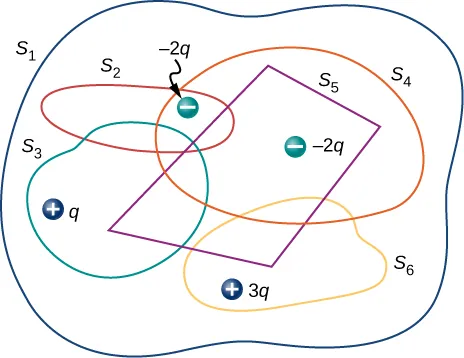31.

Find the electric flux through the closed surface whose cross-sections are shown below.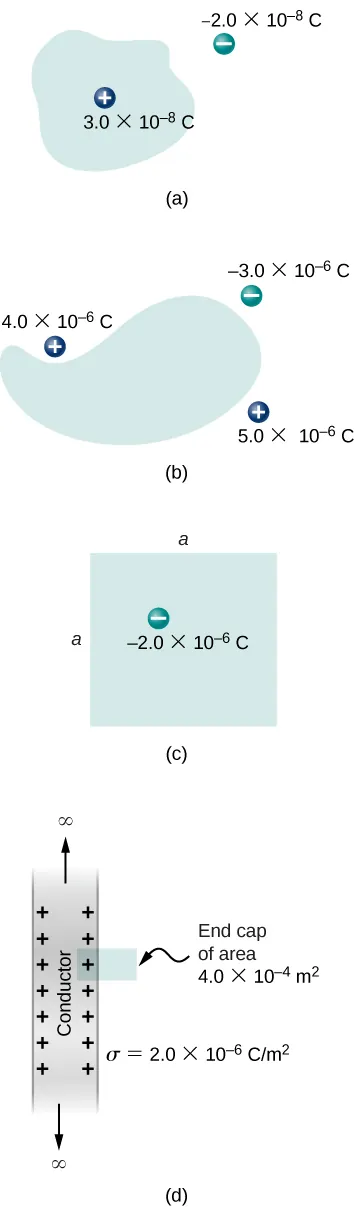32.

A point charge q is located at the center of a cube whose sides are of length a. If there are no other charges in this system, what is the electric flux through one face of the cube?

33.

A point charge of $10μC10μC$ is at an unspecified location inside a cube of side 2 cm. Find the net electric flux though the surfaces of the cube.

34.

A net flux of $1.0×104N·m2/C1.0×104N·m2/C$ passes inward through the surface of a sphere of radius 5 cm. (a) How much charge is inside the sphere? (b) How precisely can we determine the location of the charge from this information?

35.

A charge q is placed at one of the corners of a cube of side a, as shown below. Find the magnitude of the electric flux through the shaded face due to q. Assume $q>0q>0$.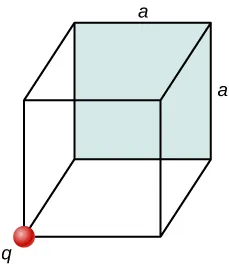36.

The electric flux through a cubical box 8.0 cm on a side is $1.2×103N·m2/C.1.2×103N·m2/C.$ What is the total charge enclosed by the box?

37.

The electric flux through a spherical surface is $4.0×104N·m2/C.4.0×104N·m2/C.$ What is the net charge enclosed by the surface?

38.

A cube whose sides are of length d is placed in a uniform electric field of magnitude $E=4.0×103N/CE=4.0×103N/C$ so that the field is perpendicular to two opposite faces of the cube. What is the net flux through the cube?

39.

Repeat the previous problem, assuming that the electric field is directed along a body diagonal of the cube.

40.

A total charge $5.0×10−6C5.0×10−6C$ is distributed uniformly throughout a cubical volume whose edges are 8.0 cm long. (a) What is the charge density in the cube? (b) What is the electric flux through a cube with 12.0-cm edges that is concentric with the charge distribution? (c) Do the same calculation for cubes whose edges are 10.0 cm long and 5.0 cm long. (d) What is the electric flux through a spherical surface of radius 3.0 cm that is also concentric with the charge distribution?

### 6.3Applying Gauss’s Law

41.

Recall that in the example of a uniform charged sphere, $ρ0=Q/(43πR3).ρ0=Q/(43πR3).$ Rewrite the answers in terms of the total charge Q on the sphere.

42.

Suppose that the charge density of the spherical charge distribution shown in Figure 6.23 is $ρ(r)=ρ0r/Rρ(r)=ρ0r/R$ for $r≤Rr≤R$ and zero for $r>R.r>R.$ Obtain expressions for the electric field both inside and outside the distribution.

43.

A very long, thin wire has a uniform linear charge density of $50μC/m.50μC/m.$ What is the electric field at a distance 2.0 cm from the wire?

44.

A charge of $−30μC−30μC$ is distributed uniformly throughout a spherical volume of radius 10.0 cm. Determine the electric field due to this charge at a distance of (a) 2.0 cm, (b) 5.0 cm, and (c) 20.0 cm from the center of the sphere.

45.

Repeat your calculations for the preceding problem, given that the charge is distributed uniformly over the surface of a spherical conductor of radius 10.0 cm.

46.

A total charge Q is distributed uniformly throughout a spherical shell of inner and outer radii $r1andr2,r1andr2,$ respectively. Show that the electric field due to the charge is

$E → = 0 → ( r ≤ r 1 ) ; E → = Q 4 π ε 0 r 2 ( r 3 − r 1 3 r 2 3 − r 1 3 ) r ^ ( r 1 ≤ r ≤ r 2 ) ; E → = Q 4 π ε 0 r 2 r ^ ( r ≥ r 2 ) . E → = 0 → ( r ≤ r 1 ) ; E → = Q 4 π ε 0 r 2 ( r 3 − r 1 3 r 2 3 − r 1 3 ) r ^ ( r 1 ≤ r ≤ r 2 ) ; E → = Q 4 π ε 0 r 2 r ^ ( r ≥ r 2 ) .$

47.

When a charge is placed on a metal sphere, it ends up in equilibrium at the outer surface. Use this information to determine the electric field of $+3.0μC+3.0μC$ charge put on a 5.0-cm aluminum spherical ball at the following two points in space: (a) a point 1.0 cm from the center of the ball (an inside point) and (b) a point 10 cm from the center of the ball (an outside point).

48.

A large sheet of charge has a uniform charge density of $10μC/m2.10μC/m2.$ What is the electric field due to this charge at a point just above the surface of the sheet?

49.

Determine if approximate cylindrical symmetry holds for the following situations. State why or why not. (a) A 300-cm long copper rod of radius 1 cm is charged with +500 nC of charge and we seek electric field at a point 5 cm from the center of the rod. (b) A 10-cm long copper rod of radius 1 cm is charged with +500 nC of charge and we seek electric field at a point 5 cm from the center of the rod. (c) A 150-cm wooden rod is glued to a 150-cm plastic rod to make a 300-cm long rod, which is then painted with a charged paint so that one obtains a uniform charge density. The radius of each rod is 1 cm, and we seek an electric field at a point that is 4 cm from the center of the rod. (d) Same rod as (c), but we seek electric field at a point that is 500 cm from the center of the rod.

50.

A long silver rod of radius 3 cm has a charge of $−5μC/cm−5μC/cm$ on its surface. (a) Find the electric field at a point 5 cm from the center of the rod (an outside point). (b) Find the electric field at a point 2 cm from the center of the rod (an inside point).

51.

The electric field at 2 cm from the center of long copper rod of radius 1 cm has a magnitude 3 N/C and directed outward from the axis of the rod. (a) How much charge per unit length exists on the copper rod? (b) What would be the electric flux through a cube of side 5 cm situated such that the rod passes through opposite sides of the cube perpendicularly?

52.

A long copper cylindrical shell of inner radius 2 cm and outer radius 3 cm surrounds concentrically a charged long aluminum rod of radius 1 cm with a charge density of 4 pC/m. All charges on the aluminum rod reside at its surface. The inner surface of the copper shell has exactly opposite charge to that of the aluminum rod while the outer surface of the copper shell has the same charge as the aluminum rod. Find the magnitude and direction of the electric field at points that are at the following distances from the center of the aluminum rod: (a) 0.5 cm, (b) 1.5 cm, (c) 2.5 cm, (d) 3.5 cm, and (e) 7 cm.

53.

Charge is distributed uniformly with a density $ρρ$ throughout an infinitely long cylindrical volume of radius R. Show that the field of this charge distribution is directed radially with respect to the cylinder and that

$E = ρ r 2 ε 0 ( r ≤ R ) ; E = ρ R 2 2 ε 0 r ( r ≥ R ) . E = ρ r 2 ε 0 ( r ≤ R ) ; E = ρ R 2 2 ε 0 r ( r ≥ R ) .$

54.

Charge is distributed throughout a very long cylindrical volume of radius R such that the charge density increases with the distance r from the central axis of the cylinder according to $ρ=αr,ρ=αr,$ where $αα$ is a constant. Show that the field of this charge distribution is directed radially with respect to the cylinder and that

$E = α r 2 3 ε 0 ( r ≤ R ) ; E = α R 3 3 ε 0 r ( r ≥ R ) . E = α r 2 3 ε 0 ( r ≤ R ) ; E = α R 3 3 ε 0 r ( r ≥ R ) .$

55.

The electric field 10.0 cm from the surface of a copper ball of radius 5.0 cm is directed toward the ball’s center and has magnitude $4.0×102N/C.4.0×102N/C.$ How much charge is on the surface of the ball?

56.

Charge is distributed throughout a spherical shell of inner radius $r1r1$ and outer radius $r2r2$ with a volume density given by $ρ=ρ0r1/r,ρ=ρ0r1/r,$ where $ρ0ρ0$ is a constant. Determine the electric field due to this charge as a function of r, the distance from the center of the shell.

57.

Charge is distributed throughout a spherical volume of radius R with a density $ρ=αr2,ρ=αr2,$ where $αα$ is a constant. Determine the electric field due to the charge at points both inside and outside the sphere.

58.

Consider a uranium nucleus to be sphere of radius $R=7.4×10−15mR=7.4×10−15m$ with a charge of 92e distributed uniformly throughout its volume. (a) What is the electric force exerted on an electron when it is $3.0×10−15m3.0×10−15m$ from the center of the nucleus? (b) What is the acceleration of the electron at this point?

59.

The volume charge density of a spherical charge distribution is given by $ρ(r)=ρ0e−αr,ρ(r)=ρ0e−αr,$ where $ρ0ρ0$ and $αα$ are constants. What is the electric field produced by this charge distribution?

### 6.4Conductors in Electrostatic Equilibrium

60.

An uncharged conductor with an internal cavity is shown in the following figure. Use the closed surface S along with Gauss’ law to show that when a charge q is placed in the cavity a total charge –q is induced on the inner surface of the conductor. What is the charge on the outer surface of the conductor?

Figure 6.46 A charge inside a cavity of a metal. Charges at the outer surface do not depend on how the charges are distributed at the inner surface since E field inside the body of the metal is zero.
61.

An uncharged spherical conductor S of radius R has two spherical cavities A and B of radii a and b, respectively as shown below. Two point charges $+qa+qa$ and $+qb+qb$ are placed at the center of the two cavities by using non-conducting supports. In addition, a point charge $+q0+q0$ is placed outside at a distance r from the center of the sphere. (a) Draw approximate charge distributions in the metal although metal sphere has no net charge. (b) Draw electric field lines. Draw enough lines to represent all distinctly different places.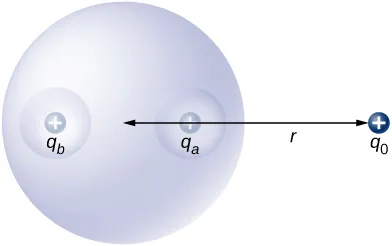62.

A positive point charge is placed at the angle bisector of two uncharged plane conductors that make an angle of $45°.45°.$ See below. Draw the electric field lines.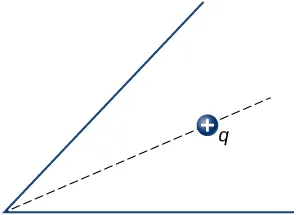63.

A long cylinder of copper of radius 3 cm is charged so that it has a uniform charge per unit length on its surface of 3 C/m. (a) Find the electric field inside and outside the cylinder. (b) Draw electric field lines in a plane perpendicular to the rod.

64.

An aluminum spherical ball of radius 4 cm is charged with $5μC5μC$ of charge. A copper spherical shell of inner radius 6 cm and outer radius 8 cm surrounds it. A total charge of $−8μC−8μC$ is put on the copper shell. (a) Find the electric field at all points in space, including points inside the aluminum and copper shell when copper shell and aluminum sphere are concentric. (b) Find the electric field at all points in space, including points inside the aluminum and copper shell when the centers of copper shell and aluminum sphere are 1 cm apart.

65.

A long cylinder of aluminum of radius R meters is charged so that it has a uniform charge per unit length on its surface of $λλ$. (a) Find the electric field inside and outside the cylinder. (b) Plot electric field as a function of distance from the center of the rod.

66.

At the surface of any conductor in electrostatic equilibrium, $E=σ/ε0.E=σ/ε0.$ Show that this equation is consistent with the fact that $E=kq/r2E=kq/r2$ at the surface of a spherical conductor.

67.

Two parallel plates 10 cm on a side are given equal and opposite charges of magnitude $5.0×10−9C.5.0×10−9C.$ The plates are 1.5 mm apart. What is the electric field at the center of the region between the plates?

68.

Two parallel conducting plates, each of cross-sectional area $400cm2400cm2$, are 2.0 cm apart and uncharged. If $1.0×10121.0×1012$ electrons are transferred from one plate to the other, what are (a) the charge density on each plate? (b) The electric field between the plates?

69.

The surface charge density on a long straight metallic pipe is $σσ$. What is the electric field outside and inside the pipe? Assume the pipe has a diameter of 2a.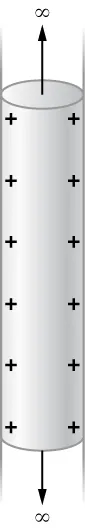70.

A point charge $q=−5.0×10−12Cq=−5.0×10−12C$ is placed at the center of a spherical conducting shell of inner radius 3.5 cm and outer radius 4.0 cm. The electric field just above the surface of the conductor is directed radially outward and has magnitude 8.0 N/C. (a) What is the charge density on the inner surface of the shell? (b) What is the charge density on the outer surface of the shell? (c) What is the net charge on the conductor?

71.

A solid cylindrical conductor of radius a is surrounded by a concentric cylindrical shell of inner radius b. The solid cylinder and the shell carry charges +Q and –Q, respectively. Assuming that the length L of both conductors is much greater than a or b, determine the electric field as a function of r, the distance from the common central axis of the cylinders, for (a) $r (b) $a and (c)$r>b.r>b.$

Order a print copy

As an Amazon Associate we earn from qualifying purchases.